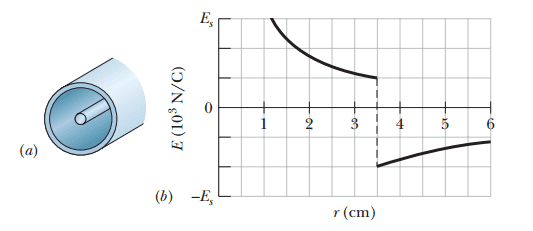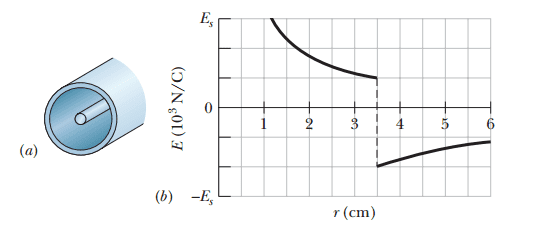# Gauss's Law problem involving a Cylinder

## Homework Statement

The figure a shows a narrow charged solid cylinder that is coaxial with a larger charged cylindrical shell. Both are non-conducting and thin and have uniform surface charge densities on their outer surfaces. Figure b gives the radial component ##E## of the electric field versus radial distance ##r## from the common axis, and ##E_{s}=3.0\times 10^{3}N/C##. What is the shell's linear charge density?## Homework Equations

##\oint \vec{E}\cdot\vec{dA}=\frac{q_{encl}}{\varepsilon_0}##

## The Attempt at a Solution

First I derived the relationship between ##E## and ##\lambda##(linear charge density), i.e.
$$\oint \vec{E}\cdot\vec{dA}=\frac{q_{encl}}{\varepsilon_0}\Longrightarrow E(2\pi rL)=\frac{\lambda L}{\varepsilon_0}\Longrightarrow E=\frac{\lambda}{2\pi r \varepsilon_0}$$
Note:Using r=3.5cm to solve everything
From the left of r=3.5cm only the field from the first cylinder will be involved so we have $$E_1=\frac{\lambda_1}{2\pi r\varepsilon_0}=1000N/C$$
Now from the right of r=3.5 using the relation ##E_T=E_1+E_2##, ##E_2=E_T-E_1=-2000-1000=-3000N/C##, then going back to the first relation,
$$E_T=\frac{\lambda_T}{2\pi r\varepsilon_0}=E_1+E_2\Longrightarrow \lambda_T=2\pi r\varepsilon_0(E_1+E_2)$$
EDIT: Okay so I was trying to calculate the wrong thing, I should be trying to find the linear charge density of the larger shell, therefore I have ##E=\frac{\lambda}{2\pi r\varepsilon_0}##, first shell: ##E_1=\frac{\lambda_1}{2\pi r\varepsilon_0}##, both shells: ##E_T=\frac{\lambda_1}{2\pi r\varepsilon_0}+\frac{\lambda_2}{2\pi r \varepsilon_0}##, ##E_T-E_c=\frac{\lambda_2}{2\pi r\varepsilon_0}##, then I have $$(E_T-E_c)2\pi r\varepsilon_0=\lambda_2$$ which gives the negative of the answer so I'm not entirely sure what went wrong.

Last edited:

ehild
Homework Helper

## Homework Statement

The figure a shows a narrow charged solid cylinder that is coaxial with a larger charged cylindrical shell. Both are non-conducting and thin and have uniform surface charge densities on their outer surfaces. Figure b gives the radial component ##E## of the electric field versus radial distance ##r## from the common axis, and ##E_{s}=3.0\times 10^{3}N/C##. What is the shell's linear charge density?## Homework Equations

##\oint \vec{E}\cdot\vec{dA}=\frac{q_{encl}}{\varepsilon_0}##

## The Attempt at a Solution

First I derived the relationship between ##E## and ##\lambda##(linear charge density), i.e.
$$\oint \vec{E}\cdot\vec{dA}=\frac{q_{encl}}{\varepsilon_0}\Longrightarrow E(2\pi rL)=\frac{\lambda L}{\varepsilon_0}\Longrightarrow E=\frac{\lambda}{2\pi r \varepsilon_0}$$
Note:Using r=3.5cm to solve everything
From the left of r=3.5cm only the field from the first cylinder will be involved so we have $$E_1=\frac{\lambda_1}{2\pi r\varepsilon_0}=1000N/C$$
Now from the right of r=3.5 using the relation ##E_T=E_1+E_2##, ##E_2=E_T-E_1=-2000-1000=-3000N/C##, then going back to the first relation,
$$E_T=\frac{\lambda_T}{2\pi r\varepsilon_0}=E_1+E_2\Longrightarrow \lambda_T=2\pi r\varepsilon_0(E_1+E_2)$$
EDIT: Okay so I was trying to calculate the wrong thing, I should be trying to find the linear charge density of the larger shell, therefore I have ##E=\frac{\lambda}{2\pi r\varepsilon_0}##, first shell: ##E_1=\frac{\lambda_1}{2\pi r\varepsilon_0}##, both shells: ##E_T=\frac{\lambda_1}{2\pi r\varepsilon_0}+\frac{\lambda_2}{2\pi r \varepsilon_0}##, ##E_T-E_c=\frac{\lambda_2}{2\pi r\varepsilon_0}##, then I have $$(E_T-E_c)2\pi r\varepsilon_0=\lambda_2$$ which gives the negative of the answer so I'm not entirely sure what went wrong.
Your derivation is correct, if Ec means E1. What result have you got?

Your derivation is correct, if Ec means E1. What result have you got?
When I plug in numbers I obtain ##\lambda_2=5.83\times10^{-9}##, the solution in the book is given as ##\lambda_2=-5.83\times10^{-9}##, another odd thing is if I solve for the values of ##\lambda## then use ##\lambda_1+\lambda_2=\lambda_t## I get the correct answer but I would think that both methods should work.

ehild
Homework Helper
When I plug in numbers I obtain ##\lambda_2=5.83\times10^{-9}##, the solution in the book is given as ##\lambda_2=-5.83\times10^{-9}##, another odd thing is if I solve for the values of ##\lambda## then use ##\lambda_1+\lambda_2=\lambda_t## I get the correct answer but I would think that both methods should work.
What is ET? Is not it negative?•Potatochip911
What is ET? Is not it negative?haha yea it appears as though I accidentally swapped the values for ##E_T## and ##E_1## when performing the calculation.